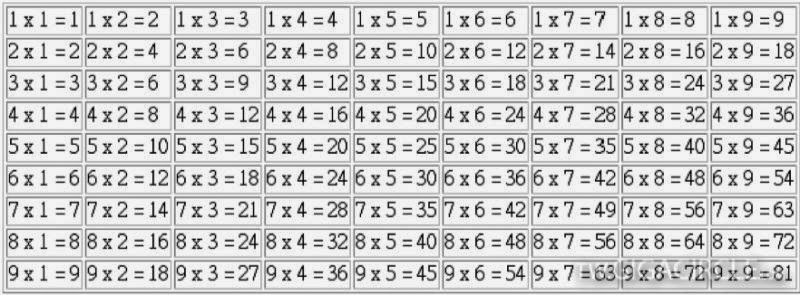Searching...
Apr 4, 2015

# 「印度式乘法」口訣秒殺「九九乘法」！趕緊告訴孩子！13 × 12 ＝ ？
(被乘數) (乘數)

13+2＝15

2×3=6

(13＋2)×10＋6＝156

14×13：
(1) 14+3＝17
(2) 17×10＝170
(3) 4×3＝12
(4) 170+12＝182

16×17：
(1) 16+7＝23
(2) 23×10＝230
(3) 6×7＝42
(4) 230+42＝272

19×19
(1) 19+9＝28
(2) 28×10＝280
(3) 9×9＝81
(4) 280+81＝361

1、 把和11相乘的數的首位和末位數字拆開，中間留出若干空位。

2、 把這個數各個數位上的數字相加，

3、 把第二步求出的和依次填寫在上一步留出的空位上。

19×11=1（1＋9）9=209

253×11=2（2+5）（5+3）3=2783

925834×11=9(9+2)(2+5)(5+8)(8+3)(3+4)4=10184174
（註：滿十進一）

1、 十位上的數字乘以比它大1的數；

2、 在上一步的得數後面緊接著寫上25

25×25= 2×（2+1）25=625

55×55= 5×（5+1）25=3025

95×95= 9×（9+1）25=9025

1、 十位上的數字乘以比它大1的數；

2、 個位數相乘

3、 將第二步的得數直接寫在步驟1的得數後面。

28×22=  2×（2+1）(8×2)=616

34×36=  3×(3+1) (4×6)=1224

67×63=  6×（6+1）（7×3）=4221

98×92=  9×（9+1）（8×2）=9016